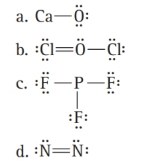# What is wrong with each Lewis structure? Fix the problem and write a correct Lewis structure.### Chemistry In Focus

7th Edition
Tro + 1 other
Publisher: Cengage Learning,
ISBN: 9781337399692

#### Solutions

Chapter
Section### Chemistry In Focus

7th Edition
Tro + 1 other
Publisher: Cengage Learning,
ISBN: 9781337399692
Chapter 5, Problem 25E
Textbook Problem
2032 views

## What is wrong with each Lewis structure? Fix the problem and write a correct Lewis structure.Interpretation Introduction

Interpretation:

The reasons for the wrong Lewis structures are to be found. The error to be fixed and the correct Lewis structure for each of the given compounds are to be written.

Concept Introduction:

The steps for drawing the Lewis structures of the ionic compounds are as follows:

The Lewis structures of elements are combined to form the Lewis structures of compounds.

The dots are transferred from the Lewis structure of the metal to the Lewis structure of the non-metal.

The metal becomes a cation and the non-metal becomes an anion.

According to the Lewis theory, in covalent bonding, atoms share their electrons. The steps for drawing a covalent Lewis structure are as follows:

Write the skeletal structure of the molecule.

Add the number of valence electrons of each atom in the molecule in order to calculate the total number of electrons present in the molecule.

Place the electrons by dots to complete the octets of the atoms.

If the central atom has not obtained an octet, then its multiple bonds can be formed.

### Explanation of Solution

a)

CaO is an ionic compound.

Calcium (Ca) and oxygen (O) have the following Lewis structures:

So, calcium loses its two valence electrons to obtain an octet, while oxygen needs two electrons to complete its octet.

Hence, the correct Lewis structure of CaO is as follows:

b) The given structure is as follows:

The given compound is a covalent compound. Hence, the electrons are shared between the atoms.

Oxygen is the central atom and chlorine atoms are arranged around it. Thus, the skeletal structure of the compound is:

Cl     O     Cl

Now, determine the total number of electrons for the molecule.

Total electrons = valence electrons for Cl + valence electrons for O= 2(7)+6= 20

These electrons are distributed in the structure to complete the octet of each atom. But, in a given molecule, chlorine has ten electrons.

So, the correct Lewis structure will be as follows:

As all the atoms in the structure have obtained an octet, the structure is complete.

c) The given structure is as follows:

### Still sussing out bartleby?

Check out a sample textbook solution.

See a sample solution

#### The Solution to Your Study Problems

Bartleby provides explanations to thousands of textbook problems written by our experts, many with advanced degrees!

Get Started

Find more solutions based on key concepts
Most people who successfully maintain weight loss do all of the following except continue to employ many of the...

Nutrition: Concepts and Controversies - Standalone book (MindTap Course List)

_______ is/are removed from a new mRNA a. Introns b. Exons c. A poly-A tail d. Amino acids

Biology: The Unity and Diversity of Life (MindTap Course List)

Why are mathematical results rounded?

An Introduction to Physical Science

List the functions of the cardiovascular system.

Human Biology (MindTap Course List)

How do the properties of seawater differ from those of fresh water?

Oceanography: An Invitation To Marine Science, Loose-leaf Versin

An unstable atomic nucleus of mass 17.0 10-27 kg initially at rest disintegrates into three particles. One of ...

Physics for Scientists and Engineers, Technology Update (No access codes included)